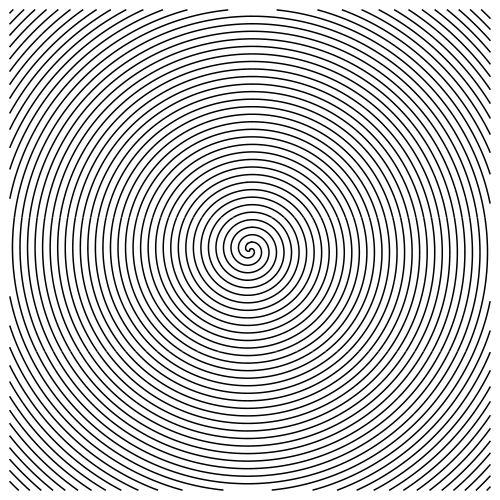6760, Math 21a, Fall 2009
Exhibits page Math 21a 2009, Multivariable Calculus
Hypnotic Spiral
 The hyptnotic spiral ``` z = cos(r+theta) ``` Appears in the iphone application PatternsLE. What does the above formula mean? As written, it is the graph of a function f(r,theta) in polar coordinates. How should this function be writen in cartesian coordinates?Here is a Mathematica implementation which generated the above picture ```ContourPlot[ Cos[Sqrt[x^2+y^2]+If[x<0,Pi,0]+ArcTan[y/x]],{x,-100,100},{y,-100,100}, Axes->False,Frame->False,ContourShading->False,Contours->{0},PlotPoints->100, ContourStyle -> {Thickness[0.003]},ImageSize->500 ] ```